# Resources tagged with: Coordinates

Filter by: Content type:
Age range:
Challenge level:

There are 52 NRICH Mathematical resources connected to Coordinates, you may find related items under Coordinates, Functions and Graphs.

Broad Topics > Coordinates, Functions and Graphs > Coordinates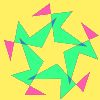### Attractive Rotations

##### Age 11 to 14Challenge Level

Here is a chance to create some attractive images by rotating shapes through multiples of 90 degrees, or 30 degrees, or 72 degrees or...### Treasure Hunt

##### Age 7 to 14Challenge Level

Can you find a reliable strategy for choosing coordinates that will locate the treasure in the minimum number of guesses?### Eight Hidden Squares

##### Age 7 to 14Challenge Level

On the graph there are 28 marked points. These points all mark the vertices (corners) of eight hidden squares. Can you find the eight hidden squares?### Route to Infinity

##### Age 11 to 14Challenge Level

Can you describe this route to infinity? Where will the arrows take you next?### Cubestick

##### Age 16 to 18Challenge Level

Stick some cubes together to make a cuboid. Find two of the angles by as many different methods as you can devise.### Square Coordinates

##### Age 11 to 14Challenge Level

A tilted square is a square with no horizontal sides. Can you devise a general instruction for the construction of a square when you are given just one of its sides?### Isosceles Triangles

##### Age 11 to 14Challenge Level

Draw some isosceles triangles with an area of $9$cm$^2$ and a vertex at (20,20). If all the vertices must have whole number coordinates, how many is it possible to draw?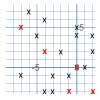### Ten Hidden Squares

##### Age 7 to 14Challenge Level

These points all mark the vertices (corners) of ten hidden squares. Can you find the 10 hidden squares?### Coordinate Patterns

##### Age 11 to 14Challenge Level

Charlie and Alison have been drawing patterns on coordinate grids. Can you picture where the patterns lead?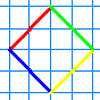### A Cartesian Puzzle

##### Age 7 to 11Challenge Level

Find the missing coordinates which will form these eight quadrilaterals. These coordinates themselves will then form a shape with rotational and line symmetry.### Parabella

##### Age 16 to 18Challenge Level

This is a beautiful result involving a parabola and parallels.### Beelines

##### Age 14 to 16Challenge Level

Is there a relationship between the coordinates of the endpoints of a line and the number of grid squares it crosses?##### Age 14 to 16Challenge Level

A 1 metre cube has one face on the ground and one face against a wall. A 4 metre ladder leans against the wall and just touches the cube. How high is the top of the ladder above the ground?### Being Collaborative - Lower Primary Geometry

##### Age 5 to 7

Geometry problems for you to work on with others.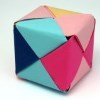### Being Resilient - Primary Geometry

##### Age 5 to 11Challenge Level

Geometry problems at primary level that may require resilience.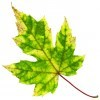### Being Resourceful - Primary Geometry

##### Age 5 to 11Challenge Level

Geometry problems at primary level that require careful consideration.### Being Collaborative - Primary Geometry

##### Age 5 to 11Challenge Level

Geometry problems for primary learners to work on with others.### Being Curious - Primary Geometry

##### Age 5 to 11Challenge Level

Geometry problems for inquiring primary learners.### Hidden Squares

##### Age 11 to 14Challenge Level

Can you find the squares hidden on these coordinate grids?### Polar Bearings

##### Age 16 to 18Challenge Level

What on earth are polar coordinates, and why would you want to use them?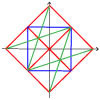### Coordinate Designs

##### Age 11 to 14Challenge Level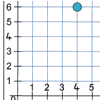### Two Number Lines

##### Age 7 to 11Challenge Level

Max and Mandy put their number lines together to make a graph. How far had each of them moved along and up from 0 to get the counter to the place marked?### Flight Path

##### Age 16 to 18Challenge Level

Use simple trigonometry to calculate the distance along the flight path from London to Sydney.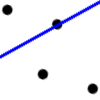### Dotty Relationship

##### Age 7 to 11Challenge Level

Can you draw perpendicular lines without using a protractor? Investigate how this is possible.### Parallel Parking

##### Age 14 to 16

Scientist Bryan Rickett has a vision of the future - and it is one in which self-parking cars prowl the tarmac plains, hunting down suitable parking spots and manoeuvring elegantly into them.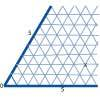### Cartesian Isometric

##### Age 7 to 11Challenge Level

The graph below is an oblique coordinate system based on 60 degree angles. It was drawn on isometric paper. What kinds of triangles do these points form?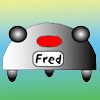### Fred the Class Robot

##### Age 7 to 11Challenge Level

Billy's class had a robot called Fred who could draw with chalk held underneath him. What shapes did the pupils make Fred draw?### 3D Treasure Hunt

##### Age 14 to 18Challenge Level

Some treasure has been hidden in a three-dimensional grid! Can you work out a strategy to find it as efficiently as possible?### Something in Common

##### Age 14 to 16Challenge Level

A square of area 3 square units cannot be drawn on a 2D grid so that each of its vertices have integer coordinates, but can it be drawn on a 3D grid? Investigate squares that can be drawn.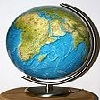### Coordinates and Descartes

##### Age 7 to 16

Have you ever wondered how maps are made? Or perhaps who first thought of the idea of designing maps? We're here to answer these questions for you.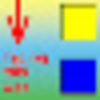### Coordinating Classroom Coordinates

##### Age 7 to 11

This article describes a practical approach to enhance the teaching and learning of coordinates.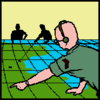### Going Places with Mathematicians

##### Age 7 to 14

This article looks at the importance in mathematics of representing places and spaces mathematics. Many famous mathematicians have spent time working on problems that involve moving and mapping. . . .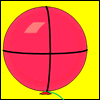### Mesh

##### Age 16 to 18Challenge Level

A spherical balloon lies inside a wire frame. How much do you need to deflate it to remove it from the frame if it remains a sphere?##### Age 14 to 16Challenge Level

Find the area of the shaded region created by the two overlapping triangles in terms of a and b?### Rational Round

##### Age 16 to 18Challenge Level

Show that there are infinitely many rational points on the unit circle and no rational points on the circle x^2+y^2=3.### Corridors

##### Age 14 to 16Challenge Level

A 10x10x10 cube is made from 27 2x2 cubes with corridors between them. Find the shortest route from one corner to the opposite corner.### Snookered

##### Age 14 to 18Challenge Level

In a snooker game the brown ball was on the lip of the pocket but it could not be hit directly as the black ball was in the way. How could it be potted by playing the white ball off a cushion?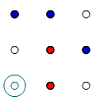### Coordinate Cunning

##### Age 7 to 11Challenge Level

A game for two people that can be played with pencils and paper. Combine your knowledge of coordinates with some strategic thinking.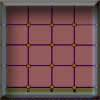### Criss Cross Quiz

##### Age 7 to 11Challenge Level

A game for 2 players. Practises subtraction or other maths operations knowledge.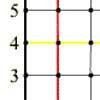### What Are You Plotting?

##### Age 7 to 11Challenge Level

Investigate the positions of points which have particular x and y coordinates. What do you notice?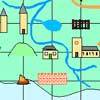### Treasure Island

##### Age 7 to 11Challenge Level

Write down what you can see at the coordinates of the treasure island map. The words can be used in a special way to find the buried treasure. Can you work out where it is?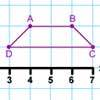### Transformation Tease

##### Age 7 to 11Challenge Level

What are the coordinates of this shape after it has been transformed in the ways described? Compare these with the original coordinates. What do you notice about the numbers?### A Tilted Square

##### Age 14 to 16Challenge Level

The opposite vertices of a square have coordinates (a,b) and (c,d). What are the coordinates of the other vertices?### Square Pair Circles

##### Age 16 to 18Challenge Level

Investigate the number of points with integer coordinates on circles with centres at the origin for which the square of the radius is a power of 5.### Hypotenuse Lattice Points

##### Age 14 to 16Challenge Level

The triangle OMN has vertices on the axes with whole number co-ordinates. How many points with whole number coordinates are there on the hypotenuse MN?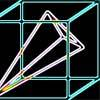### Four Points on a Cube

##### Age 16 to 18Challenge Level

What is the surface area of the tetrahedron with one vertex at O the vertex of a unit cube and the other vertices at the centres of the faces of the cube not containing O?### Plane to See

##### Age 16 to 18Challenge Level

P is the midpoint of an edge of a cube and Q divides another edge in the ratio 1 to 4. Find the ratio of the volumes of the two pieces of the cube cut by a plane through PQ and a vertex.### Napoleon's Hat

##### Age 16 to 18Challenge Level

Three equilateral triangles ABC, AYX and XZB are drawn with the point X a moveable point on AB. The points P, Q and R are the centres of the three triangles. What can you say about triangle PQR?### Cushion Ball

##### Age 16 to 18Challenge Level

The shortest path between any two points on a snooker table is the straight line between them but what if the ball must bounce off one wall, or 2 walls, or 3 walls?### Just Opposite

##### Age 14 to 16Challenge Level

A and C are the opposite vertices of a square ABCD, and have coordinates (a,b) and (c,d), respectively. What are the coordinates of the vertices B and D? What is the area of the square?